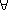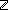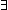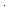IF YOU CAN SEE THIS go to /sfa/Nuprl/Shared/Xindentation_hack_doc.html
The q and r computed by the extracts of these theorems are not the same as those returned by the "a | b" and "a rem b" built-in arithmetic functions: with those functions, the sign of r follows the sign of a and div has simple symmetry properties.
Here, things are set up so that the same inequalities for r are satisfied over 2 adjacent quadrants of a,b: r is closer to the `mod' function.
This makes Thm*a,b:y:. GCD(a;b;y)
and Thm*a,b:u,v:. GCD(a;b;ua+vb)
simpler to prove, since two quadrants can be easily taken care of on the first induction.